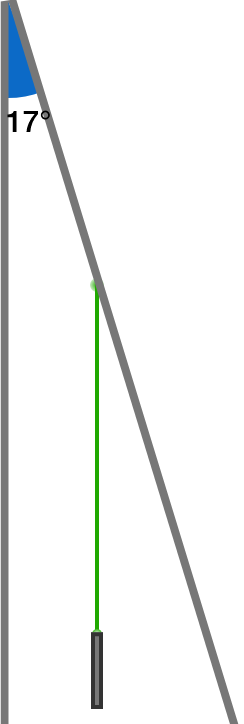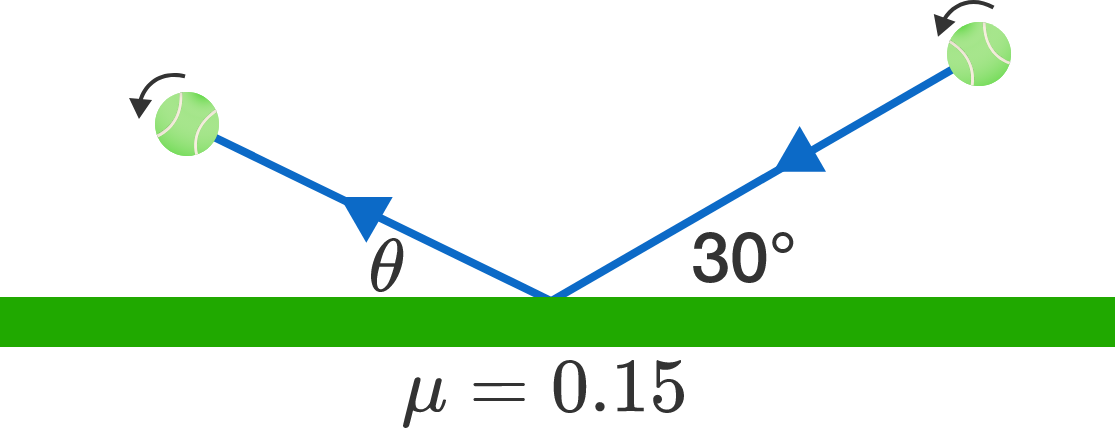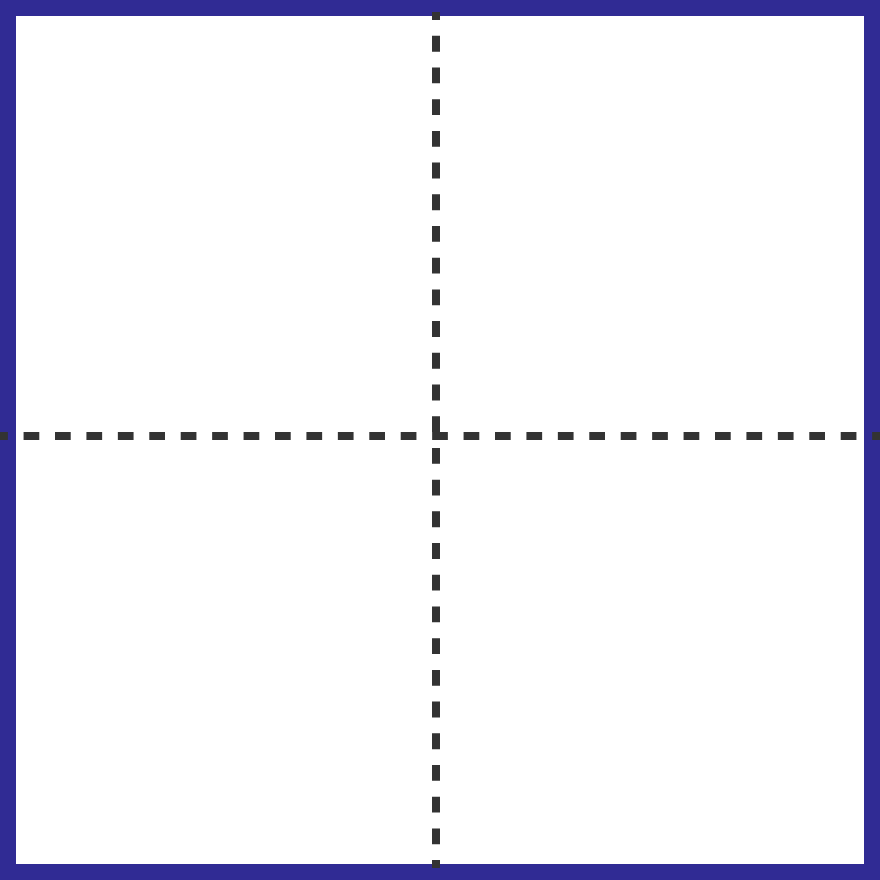# Problems of the Week

Contribute a problem

# 2017-09-11 Intermediate

Which is larger?

\begin{aligned} A &= \sqrt{2} + \sqrt{4} + \cdots + \sqrt{98} + \sqrt{100} \\\\ B &= \sqrt{1} + \sqrt{3} + \cdots + \sqrt{97} + \sqrt{99} + \sqrt{101} \end{aligned}Two plane mirrors (shown to the right) both stand perpendicular to the floor and meet at the angle $\theta = 17^\circ.$ A laser beam is pointed at one of the mirrors such that the beam travels parallel to the other mirror and to the floor.

At how many points will the light be reflected by the two mirrors?


Clarification: The figure shows the top view of the two mirrors and the laser beam.

A tennis ball hits the court with angle of incidence $30^\circ,$ and the ball has such a perfect top spin that even after it bounces, it is still spinning fast.

At what angle (in degrees relative to the horizontal) will the ball emerge after the collision?Details and Assumptions:

• A top spin means that the top of the ball moves in the same direction as the center of the ball, while the bottom of the ball moves in the opposite direction. For a really good top spin, the rotation of the ball is so fast that $\omega r \gg v$ is satisfied $(\omega$ is the angular velocity of the ball, $r$ is the ball's radius, and $v$ is the ball's velocity$).$
• Assume that the collision is as elastic as possible. The deformation of the ball during the collision does not take away any kinetic energy from the ball. On a perfectly slippery court, the ball would bounce back with the same kinetic energy.
• The coefficient of friction between the ball and the surface of the court is $0.15$.

I've partitioned a unit square into 4 rectangles and each rectangle has perimeter 2, as illustrated in the diagram. I can also cut this unit square into all of the following numbers of rectangles, each with perimeter 2, except for one number.

Which one is it?A small ball undergoes a partially elastic collision with a rough horizontal surface. Assume that friction $f=\mu N$ during the contact period, where $N$ is the normal reaction and $\mu$ is the coefficient of friction.

Find the value of the incoming angle $\theta$ (in degrees) such that the horizontal range of the ball after hitting the surface is maximized.


Details and Assumptions:

• Take the coefficient of friction $\mu = \frac{1}{\sqrt{3}}.$
• Take the coefficient of restitution $e=\sqrt{3}-1$.

Inspired from Rajdeep Brahma

×